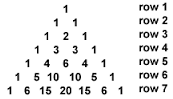# Pascal's odd rows

These are the first few rows of Pascal's triangle:Each number is derived by adding up the two numbers just above it (and to the left and right) in the previous row. (The numbers on the ends remain 1).

Of the first 1000 rows, as labeled above, how many of them contain all odd numbers?

×